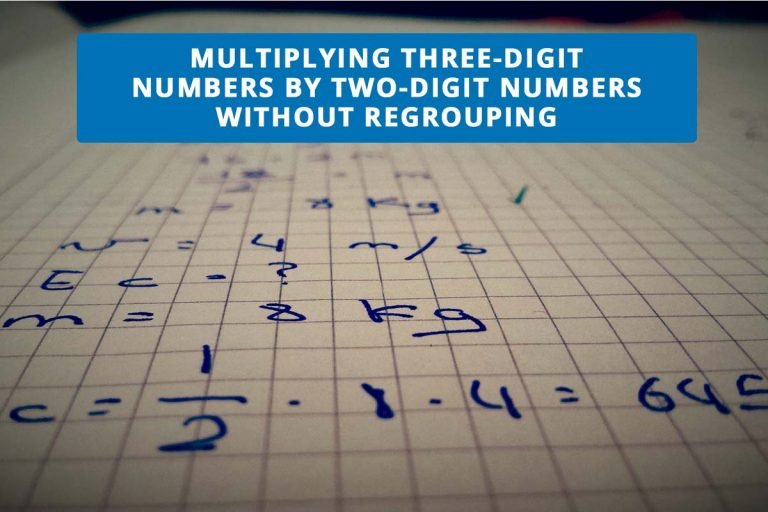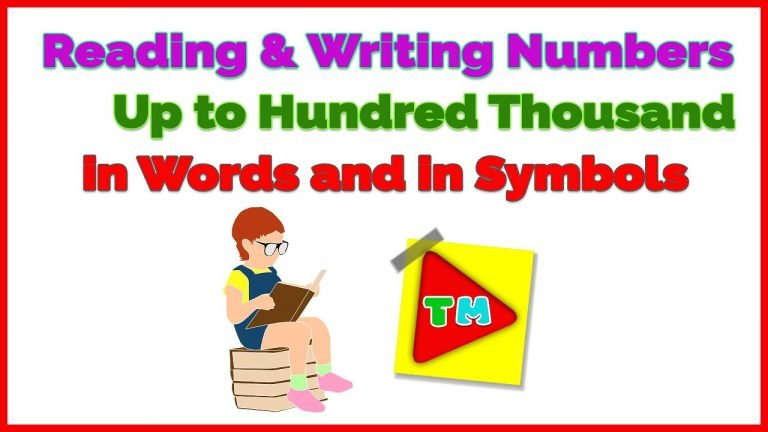﻿ September 2021 | Zest Say# Month September 2021

## MULTIPLYING THREE-DIGIT NUMBERS BY TWO-DIGIT NUMBERS WITHOUT REGROUPINGIn multiplying three-digit numbers by two-digit numbers without regrouping, follow these steps.Step 1:           Multiply the multiplicand by the ones in the multiplier.Step 2:           Multiply the multiplicand by the tens in the multiplier.Step 3:           Add the partial products. For further explanation…

## Visualizing Numbers Up to 100 000 Using Number DiscsTo visualize numbers up to 100 000, we can use number discs. Visualizing Numbers Using Number Discs Visualizing Numbers Up to 100 000 With Emphasis on 10 001 to 100 000 Writing Numbers Using Number Discs Visualize Numbers Place Value…

## Reading and Writing Numbers Up to Hundred Thousand in Words and in SymbolsReading and Writing Numbers Up to Hundred Thousand in Words and in Symbols Writing Numbers in Words How do we write the number word? Writing Numbers in Symbols How do we write numbers in symbols? To learn more about Reading…

## ROUNDING OFF NUMBERS TO THE NEAREST TEN THOUSANDS

RULE IN ROUNDING NUMBERSWe round a number up if the digit we are rounding is followed by 5, 6, 7, 8, or 9.We round a number down if the digit we are rounding is followed by 4, 3, 2, 1,…